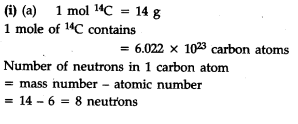# Find (a) the total number and (b) total mass of neutrons in 7 mg of \${}^{14}C\$. (Assume that mass of a neutron = 1.675 x \${{10}^{-27}}\$ kg)

(i) Find (a) the total number and (b) die total mass of neutrons in 7 mg of \${}^{14}C\$. (Assume that mass of a neutron = 1.675 x \${{10}^{-27}}\$ kg)
(ii) Find (a) the total number and (b) the total mass of protons in 34 mg of N\${{H}_{3}}\$ at STP. (Mass of 1 p = 1.6726 X \${{10}^{-27}}\$ kg) will the answer change if the temperature and pressure are changed ?